# An Example of ‘Date tool’ dimension(calculation-Dimension)

,

Recently one of my clients would like to see their Revenue figures represented some of the time as a positive (+VE) value and some time as a negative (-VE) value. They would like to have the ability to select between the two at run time in the Analyses Services Cube. The users are using Excel 2007 as the client tool for reporting. They do not want to change the figures inside the source database but would instead like to apply a mask at runtime. There are some specific post codes which are dedicated to Revenue; this enables revenue transactions to be indentified

CREATING THE DIMENSION
In order to achieve this I have created a dummy dimension, similar to a ‘time utility dimension' (also known as a ‘date tool' dimension or a ‘shell' dimension. I had advice from Chris Webb regarding this, he also referred me the book ‘Expert Cube Development with SQL Server Analysis Services 2008') and then add a calculated member to it with two values.

We do not need to create a table in our Data warehouse for this purpose; we can create a view some thing like this

CREATE VIEW RevenueAsPositive AS
SELECT 0 AS ID, 'Convert Revenue to Positive' AS Description
UNION ALL
SELECT 1 AS ID, 'Stay Revenue as Negative' AS Description
Now we need to add this view inside our DSV and create a dimension based on this view
Alternatively we can create some thing like this inside our DSV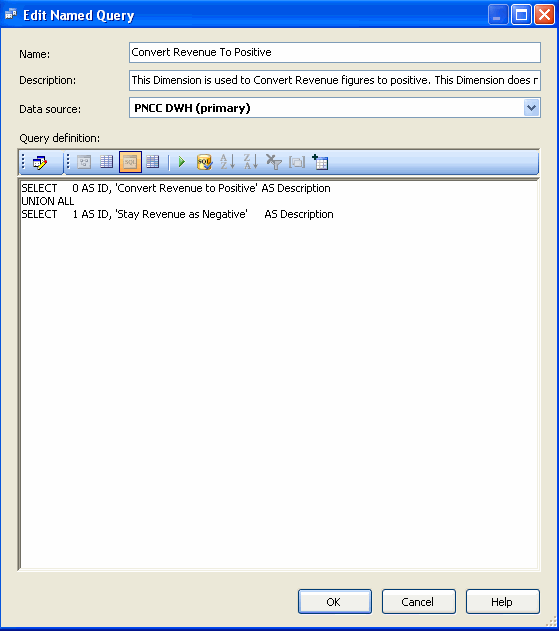So this dimension has two values

1. Convert Revenue to Positive --> 0
2. Stay Revenue as Negative --> 1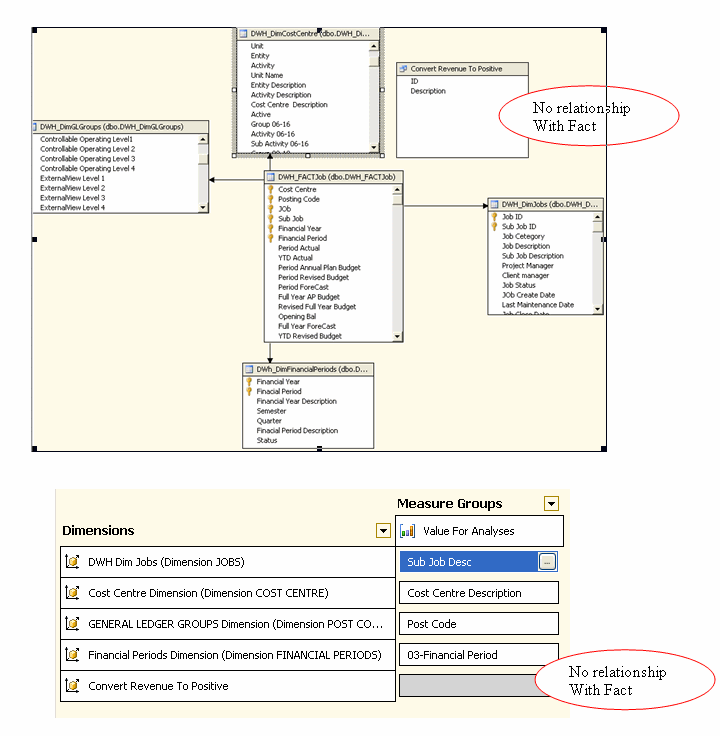CONFIGURING THE DIMENSION

After creating this dimension now you need to set the properties of the attribute hierarchy

• Set "DefaultMember" to ‘Stay Revenue as Negative', as below: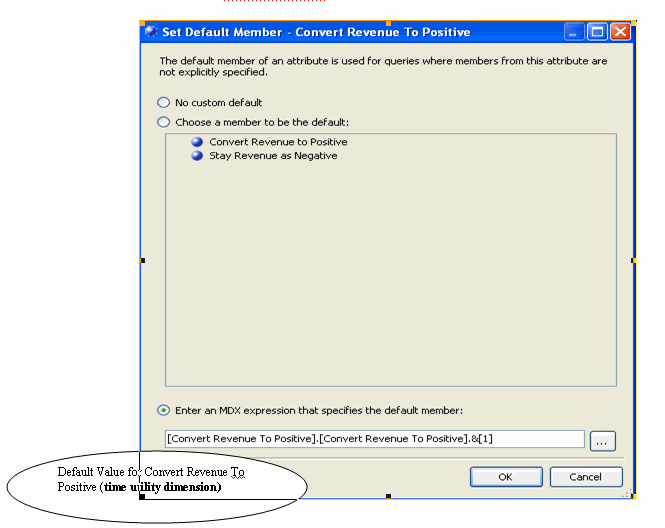•Set the “IsAggregatable” property to “False”, so there’s no All Member on the attribute hierarchy, as below: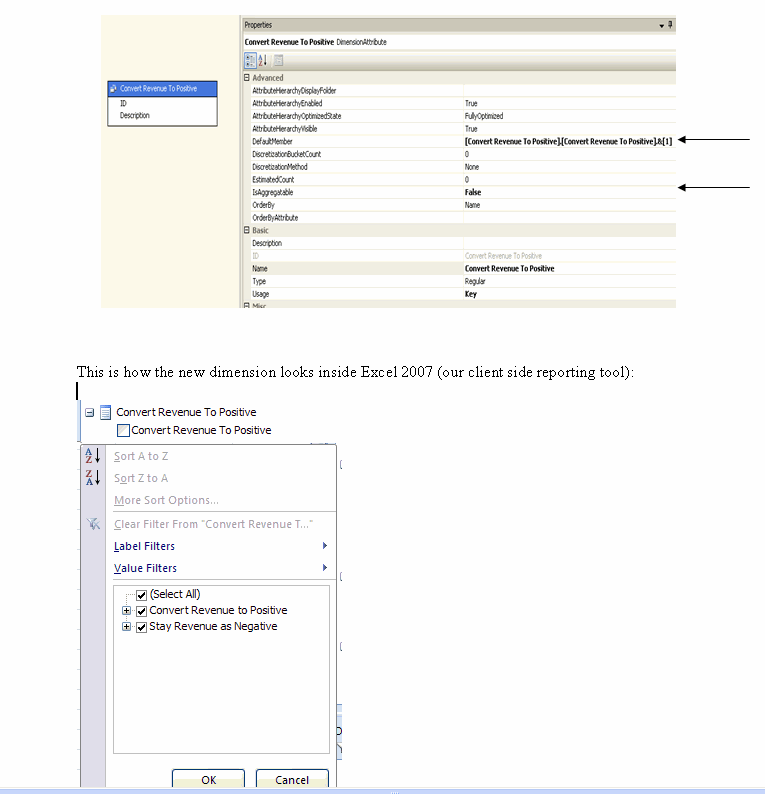In the following picture you can see all the revenue figures are negative, which is the default behavior we have defined inside the Analyses Services Cube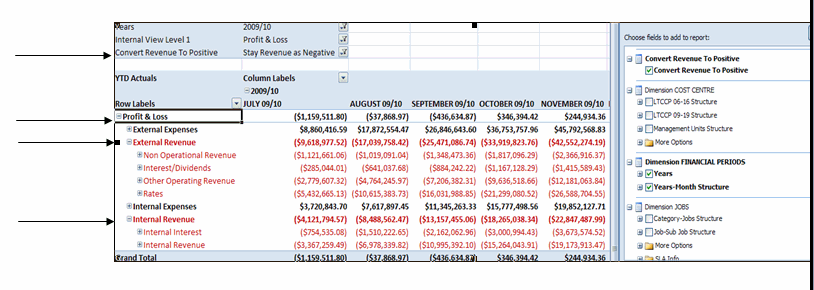If now the user would like to convert the revenue figures to display as positive, they need to set the report filter to Convert Revenue to Positive as shown in the following figure: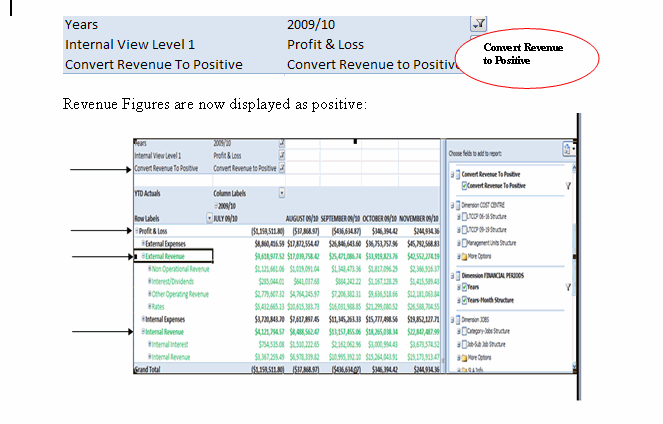In order to achieve this functionality you need to use a scoped assignment using MDX that's something like this:

When the user chooses "Convert Revenue to Positive" than I multiplied all actual measure values by -1, using this MDX:

SCOPE([Convert Revenue To Positive].[Convert Revenue To Positive].&amp;,descendants([Dimension POST CODE].[Post Code Structure].[PC Level 2].&amp;[IREV],[Dimension POST CODE].[Post Code Structure].[Post Code],self_and_before),[Measures].[YTD Actuals]
);
This =(([Dimension POST CODE].[Post Code Structure].currentmember)*(-1));
END SCOPE;

SCOPE([Convert Revenue To Positive].[Convert Revenue To Positive].&amp;,descendants([Dimension POST CODE].[Post Code Structure].[PC Level 2].&amp;[EREV],[Dimension POST CODE].[Post Code Structure].[Post Code],self_and_before),[Measures].[YTD Actuals]
);
This =(([Dimension POST CODE].[Post Code Structure].currentmember)*(-1));
END SCOPE;

Notice that the "Profit and Loss" values do not change even though the Revenue values have changed to positive. This is achieved through the following SCOPE statement:

SCOPE([Convert Revenue To Positive].[Convert Revenue To Positive].&amp;,[Dimension POST CODE].[Post Code Structure].[PC Level 1].&amp;[PL],[Measures].[YTD Actuals]
);
This =(([Dimension POST CODE].[Post Code Structure].&amp;[EEXP])+[Dimension POST CODE].[Post Code Structure].&amp;[IEXP]-[Dimension POST CODE].[Post Code Structure].&amp;[IREV]-[Dimension POST CODE].[Post Code Structure].&amp;[EREV]);
END SCOPE;

Hopefully this will help you if you are trying to build some similar functionality.

Finally, I would like to acknowledge and thank Chris Webb for his suggestions and support, thank you Chris.